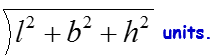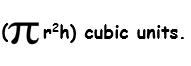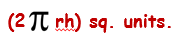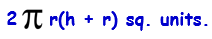# Volume and Surface Area Quantitative Aptitude Interview Questions and Answers Part 1

## 1.Cuboid Volume and Area:

• Volume = (l x b x h) cubic units.
• Surface area = 2(lb + bh + lh) sq. units.
• Diagonal =## 2.Cube Volume and Area:

• Volume = a3 cubic units
• Surface area = 6a2 sq. units.
• Diagonal = 3a units.

## 3.Cylinder Volume and Area:

• Volume =• Surface area =• Total Surface Area =